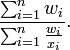### Seeing is believing!

Before you order, simply sign up for a free user account and in seconds you'll be experiencing the best in CFA exam preparation.

##### Subject 12. Valuation Indicators: Issues in Practice
Averaging Multiples: The Harmonic Mean

Analysts often use the harmonic mean and the weighted harmonic mean to average a group of multiples. The harmonic mean is the reciprocal of the arithmetic mean of the reciprocals.

X = n/(Σ1/Xi)

If a set of weight w1, w2, ..., wn is associated to the dataset x1, x2, ..., xn, the weighted harmonic mean defined by:The harmonic mean is the preferable method for averaging multiples, such as the price/earning ratio, in which price is in the numerator. If these ratios are averaged using an arithmetic mean (a common error), high data points are given greater weights than low data points. The harmonic mean, on the other hand, gives equal weight to each data point.

In general the harmonic mean is less than the arithmetic mean.

The Use of Stock Screens in Investment Management

Screening is the application of a set of criteria to reduce an investment universe to a smaller set of investments and is a part of many stock selection disciplines. In general, limitations of such screens include the lack of control in vendor-provided data of the calculation of important inputs (such as EPS) and the absence of qualitative factors.

User Comment
rhardin Can anybody help me out with an example of the weighted harmonic mean? Thanks.
tim2 Example of weighted mean. You have two companies one with a PE of 10, one 20,
the unweighted harmonic mean is
((1/10+1/20)/2)^-1

Say the PE 10 company was twice the size you could weight the average by market cap =
((2*1/10+1/20)/3)^-1
rhardin thank you tim2, I understand now!
davidt876 I found Prof. Mohanty's explanation for why we use the harmonic mean really good. similar to the argument for why you should use the geometric mean for averaging returns.